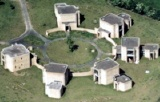# Applied Mathematics Seminar Abstracts

## Spring Term 2008/09

Wednesday 7 January 2009
Mark Chaplain
(Dundee) Multiscale mathematical modelling of cancer growth

Wednesday 21 January 2009
S.J. Chapman
(Oxford) Stochastic models for reaction-diffusion

We consider two different position jump processes: (i) a random walk on a lattice (ii) the Euler scheme for the Smoluchowski differential equation. Both of these reduce to the diffusion equation as the time step and size of the jump tend to zero. We consider the problem of adding chemical reactions to these processes, both at a surface and in the bulk. We show how the "microscopic" parameters should be chosen to achieve the correct "macroscopic" reaction rate. This choice is found to depend on which stochastic model for diffusion is used.

Wednesday 28 January 2009
Jose Rodrigo (Warwick) Singularities for aggregation equations with fractional dissipation

Wednesday 4 February 2009
Takashi Sakajo (Hokkaido) Blow-up and global solutions for systems related to the Constantin-Lax-Majda equation

It is one of the most difficult mathematical problems to show existence and uniqueness of solutions to the Navier-Stokes (NS) equations for the 3D incompressible fluids. The NS equations contain two nonlinear terms, which are referred to as the vortex-stretching term and the advection(convection) term. Since the blow-up of solutions for the 3D NS equations is said to be caused by the vortex-stretching term, Constantin, Lax and Majda consider a one-dimensional model equation of the 3-D Euler equations for non-viscous and incompressible fluids in order to understand the effect of the vortex-stretching term, which is known as Constantin-Lax-Majda (CLM) equation. Most of the solution of the CLM equation blow up in finite time and thus it successfully describes the growing mechanism of the vorticity for the 3D equations. However, on the other hand, the CLM equation contains neither advection term nor viscous term. Thus we further need to see how these terms could affect the growth of the vorticity in the CLM equation, with which we can understand the balance of the nonlinear and linear terms in the 3D NS equations. In the present talk, I will introduce two extensions for the CLM equation, one with a viscous term and one with an advection term, and I present some mathematical results on the global existence of solutions to these equations. The vortex-stretching term is so strong that viscous term cannot control the blow up when the viscous coefficient is small, while the advection term, which has been considered to have less influence on the growing-up mechanism of the vorticity than the vortex stretching term does, allows the CLM equation to have a global smooth solution. This indicates the advection term plays more important roles to the existence of global solution than we imagine.

Wednesday 11 February 2009
Johannes Zimmer (Bath) tbc

Wednesday 18 February 2009
Björn Stinner (Warwick) On a diffuse interface method for PDEs on surfaces

A finite element approach to solve a diffusion equation on a moving surface represented by a thin layer will be presented. A suitable bulk density approximating the surface as measure is employed to formulate the problem. Issues to be discussed are solvability of the degenerate bulk equation, convergence as the layer thickness tends to zero, solvability and stability of the discrete system, and mass conservation of the numerical method. Towards applications, free boundary problems involving surface quantities subject to surface pdes may be modelled using the phase field methodology which naturally leads to thin interfacial layers. Exemplary, we will consider surface active agents on the interface in two-phase flow described by a Cahn-Hilliard-Navier-Stokes system.

Wednesday 25 February 2009
Isaac Vikram Chenchiah (Bristol) Frustration in polycrystals

Wednesday 4 March 2009
Gerd Dziuk (Freiburg) Numerical methods for geometric evolution equations

Wednesday 11 March 2009
Assyr Abdulle (EPFL) Computational methods for multiscale PDEs: modeling, analysis and simulationInternet Access at Warwick:
Where possible, visitors should obtain an EDUROAM account from their own university to enable internet access whilst at Warwick.
If you need WiFi whilst at Warwick, click here for instructions (upon arrival at Warwick)
Registration:
You can register for any of the symposia or workshops online. To see which registrations are currently open and to submit a registration, please click hereLink opens in a new window.
Contact:
Mathematics Research Centre
Zeeman Building
University of Warwick
Coventry CV4 7AL - UK
E-mail:
MRC@warwick.ac.uk